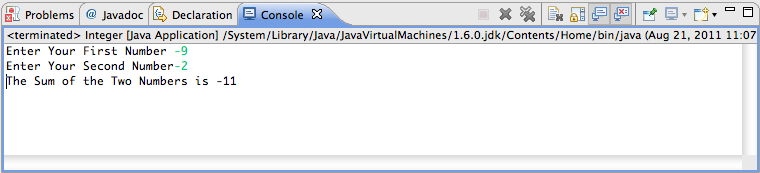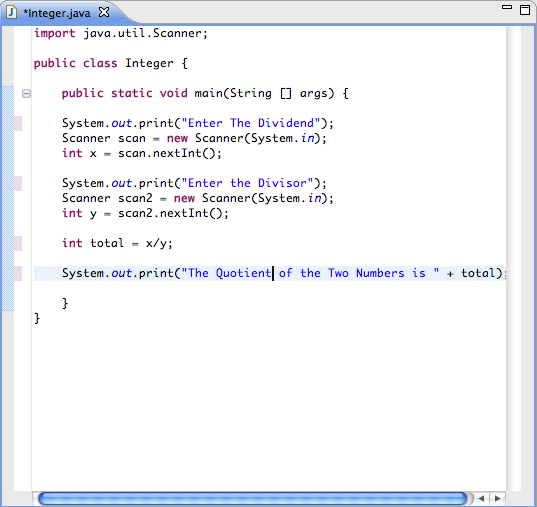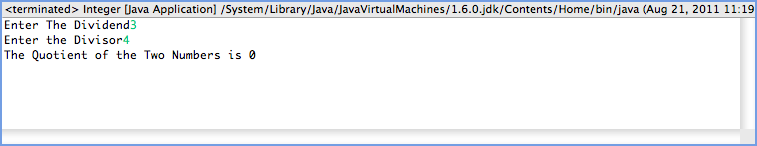# JAVA Tutorial II – Data Types (Part II – Integers)

In this tutorial, we’ll create simple programs using Eclipse that compute solid numbers. We’ll create a program the divides 2 numbers and a program that adds 2 numbers.

What’s an Integer?

An integer is a number that can be expressed as either a negative or a positive value, but can never be a decimal value. For instance, 3.4 is not an integer, but -122 is an integer.

Ex) 4/2 = 2. The program will respond with a 2.

Ex) 4/3 = 1.33333… The program will respond with a 1 instead of the expected/correct answer of 1.33333 .

Why? Because you specified the number to be an Integer, not a Float or Double.

Setting Up the Project

I’m assuming you are familiar with setting up a standard Java Program in Eclipse. But in case it slipped your memory here are the steps:

1. Go to File > New > Java Project

2. Label the project “Integer”

3. Go to File > New > Class

4. Click Browse adjacent to source and select “Integer” as the Source folder

5. Label the class “Integer”

Congratulations!

Part I of Part II of Java Tutorial II

So we’ll start with observing the adding of integers in a Java program. Here is the code you’ll need:

 import java.util.Scanner;public class Integer {public static void main(String [] args) {

System.out.print(“Enter Your First Number”); Scanner scan = new Scanner(System.in); int x = scan.nextInt(); System.out.print(“Enter Your Second Number”); Scanner scan2 = new Scanner(System.in); int y = scan2.nextInt(); int total = x+y; System.out.print(“The Sum of the Two Numbers is ” + total); } } If you want to look at a colored example as the text may help you see the code better, here is a screenshot of the code. import java.util.Scanner; There are 2 major ways of allowing the user to input data. You can use BufferedReader (which we will eventually cover) or Scanner. Scanner is much easier to use and for our learning sake, we’ll use it. But in order to use the Scanner, you have to import it first. import java.util.Scanner; is the piece of code you use. So when you use the scanner, you do the following: 1. Scanner scan = new Scanner(System.in); //System.in means you are going to input data. “scan” after Scanner is like a new instance of it. That’s why during the second block of code, you use “scan2” because scan is already in use. 2. int x = scan.nextInt(); //You declare the integer you are using here and you MUST put the “scan” that you are going to scan with. This is very vague isn’t it? Well, you can figure this part out if my explanation was too obscure (chances are it was) Declaring Ints You can declare an integer anywhere by placing the int next to a name followed by a semi-colon. But in this case, it has to be declared at a certain point. And that certain point is directly after you declare the Scanner. Addition Logic When adding 2 numbers, you have to come up with the resulting integer. “Int total = x+y;” was the code to use.Example of the Console's Building of the Application Part II of Part II of Java Tutorial II Now we are going to look at division. Not because division is cool or difficult or starts with a “d.” Division requires a float to be as the quotient of the problem because most division problems result with a non-integral number. But since this is an Integer tutorial, we are going to not use float just because we are rebels. Creating the Program You do not need to create a new class or anything. Just stick with what you already have. All you have to do is change the section where it says “int total = x+y” to “int total = x/y.” Then you can change all of the System.out.print to (“Enter the Dividend”) and to (“Enter the Divisor”). Then you can change the System.out.print that says (“The Sum of the Two Numbers is” + total); to (“The Quotient of the Two Numbers is” + total); Here is what the code should look like in the program:Notice the changes in the System.out.print and the Math Logic So let’s compile this program and launch it. If you enter numbers that would normally result in a decimal, it’ll round down. Always. So you should replace the “Int” before “total” with a “Float.”Just What I thought. 4 divided by 3 equals 0. I'll ace AP Division for sure. So, you are now a master of inputting floats and such. Congratulations. Are you ready for Part III, floats? – Next Page>> <

Tags:

Categories:

Updated: